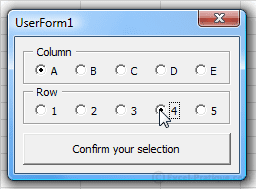# VBA Course: Controls

Controls have all sorts of properties, and the events associated with them vary, but for now we will only look at a few of the many possible uses of controls in VBA coding.

Let's start by adding the following 3 controls: a Label, a TextBox and a CommandButton: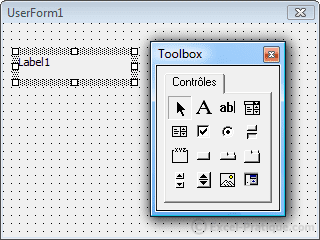Now let's edit the names and properties of the controls (using the Caption property, which contains the text). We want the following result: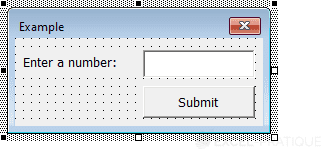For now, when we enter a number and press OK, nothing happens.

To make something happen, we'll start by adding an event that puts the value of the text box into cell A1 and closes the UserForm.

You can access the options that you see immediately below this text by double clicking on a control: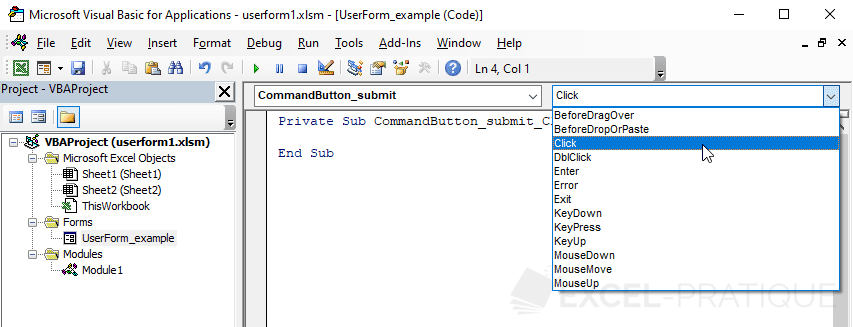The drop-down list contains different controls and the UserForm.

Select a button and an event Click:

``````Private Sub CommandButton_validate_Click()

Range("A1") = Textbox_number.Value
'Textbox_number is the name of the text box
'Value is the property that contains the value of the text box

'We are using Me in place of the name of the UserForm (because this code is within the UserForm that we want to close)
End Sub``````

The value will now be saved in cell A1 before the closing of the UserForm.

Add a second Label and edit the following properties: Caption, Forecolor (color: red) and Visible (False, to hide the control by default):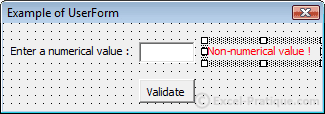Now let's add an event that will be fired when the value of the text box is changed, which will display an error message if the value is not numerical.

``````Private Sub Textbox_number_Change()
If IsNumeric(Textbox_number.Value) Then 'IF numerical value ...
Label_error.Visible = False 'Label hidden
Else 'OTHERWISE ...
Label_error.Visible = True 'Label shown
End If
End Sub``````

The value will be tested each time a character is entered ...

We still need to prevent the validation of the form if the value is not numerical:

``````Private Sub CommandButton_validate_Click()
If IsNumeric(Textbox_number.Value) Then 'IF numerical value ...
Range("A1") = Textbox_number.Value 'Copy to A1
Else 'OTHERWISE ...
MsgBox "Incorrect value"
End If
End Sub``````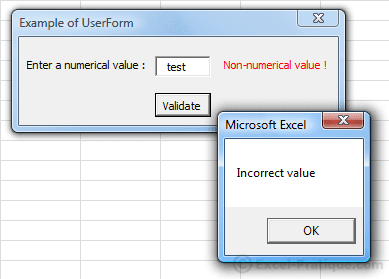So as not to leave the right-hand side of the UserForm blank when there isn't any error, we can reduce its size by modifying the UserForm's Width property:

``````Private Sub Textbox_number_Change()
If IsNumeric(Textbox_number.Value) Then 'IF numerical value ...
Label_error.Visible = False 'Label hidden
Me.Width = 156 'UserForm Width
Else 'OTHERWISE ...
Label_error.Visible = True 'Label shown
Me.Width = 244 'UserForm Width
End If
End Sub``````

See result below: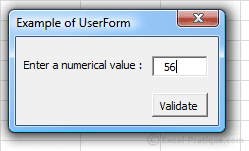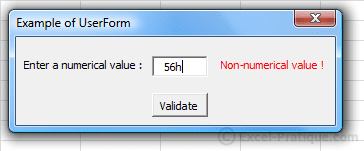## Checkboxes

Here is an example of how to use the CheckBox: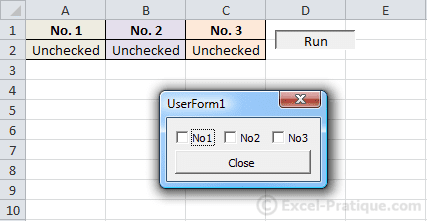When a checkbox is checked/unchecked, the value of the corresponding cell can be modified by using the Click event:

``````Private Sub CheckBox1_Click() 'Number 1
If CheckBox1.Value = True Then 'If checked ...
Range("A2") = "Checked"
Else 'If not checked ...
Range("A2") = "Unchecked"
End If
End Sub

Private Sub CheckBox2_Click() 'Number 2
If CheckBox2.Value = True Then 'If checked ...
Range("B2") = "Checked"
Else 'If not checked ...
Range("B2") = "Unchecked"
End If
End Sub

Private Sub CheckBox3_Click() 'Number 3
If CheckBox3.Value = True Then 'If checked ...
Range("C2") = "Checked"
Else 'If not checked ...
Range("C2") = "Unchecked"
End If
End Sub``````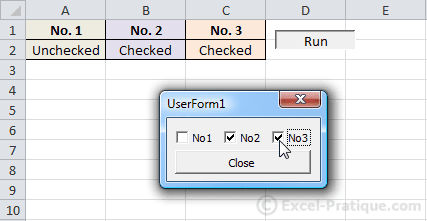In this example, the checkboxes start out unchecked when the UserForm is first opened.
To check the boxes when the value of the corresponding cell is "Checked", we'll run a test when the UserForm is activated, using UserForm_Initialize:

``````Private Sub UserForm_Initialize() 'Check box if "Checked"
If Range("A2") = "Checked" Then
CheckBox1.Value = True
End If

If Range("B2") = "Checked" Then
CheckBox2.Value = True
End If

If Range("C2") = "Checked" Then
CheckBox3.Value = True
End If
End Sub``````

## The Option Buttons

The user may only select one Option Button per "group", which is not the case with checkboxes.

To create a group, first insert a Frame and then OptionButton: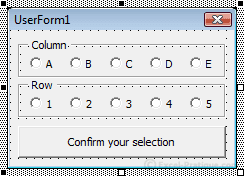Once the form has been submitted, we will enter data into the cell that corresponds to the column numbers and row_value chosen.

In order to know which option button was chosen, we could do the same as in the previous example (with the checkboxes) but we will do it with a loop to reduce the length of the code.

We're going to use a For Each loop this time, a kind of loop that we haven't yet introduced. This kind of loop makes it possible to execute instructions for each object in an "object group":

``````Private Sub CommandButton1_Click()
Dim column_value As String, row_value As String

'Loop for each Frame_column control
For Each column_button In Frame_column.Controls
'If the value of the control  = True (then, if checked) ...
If column_button.Value Then
'The variable "column_value" takes the value of the button text
column_value = column_button.Caption
End If
Next

'Loop for the other frame
For Each row_button In Frame_row.Controls
If row_button.Value Then
row_value = row_button.Caption
End If
Next

Range(column_value & row_value) = "Cell chosen !"
End Sub``````

Now this form enters the value "Cell chosen !" into the cell that has been chosen (provided that the form is complete).

To avoid a bug, we need to check to make sure that the user has chosen correctly from the two sets of option buttons.

In this example, when the form is incomplete, the "Confirm" button will appear in gray (deactived). This is not the simplest solution, but it's a good example of why functions/procedures are useful within a UserForm.

Edit the text as well as the Enabled property to deactivate the button: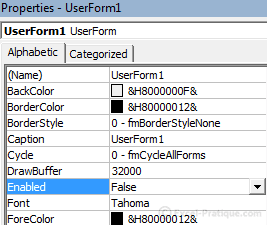The result will be: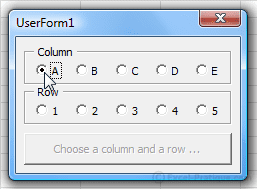In the preceding code, we used 2 For Each loops to retrieve the values of the option buttons. We will now need to use these same values for the "Confirm" button and the Click events for the ten option buttons.

So that we don't have to copy the loops for each event, we'll call them using a function.

Starting from the preceding code and modifying it, we will achieve this result:

``````Private Function column_value()
'The function returns the value of the text for the button chosen (column_value)
For Each column_button In Frame_column.Controls
If column_button.Value Then
column_value = column_button.Caption
End If
Next
End Function

Private Function row_value()
'The function returns the value of the text for the button chosen (row_value)
For Each row_button In Frame_row.Controls
If row_button.Value Then
row_value = row_button.Caption
End If
Next
End Function

Private Sub CommandButton1_Click() 'Action that is taken when you click "Confirm your selection"
Range(column_value & row_value) = "Cell chosen !"
'column_value and row_value are the values returned by the functions
End Sub``````

All we have left to do is to create a procedure that verifies that the buttons have been checked correctly (by calling the two functions), and which will activate the button if necessary.

Here again, the test is performed in a separate procedure to avoid copying the code 10x for each of the option button events:

``````Private Sub activate_button()
'Activating the button if the condition is verified
If column_value <> "" And row_value <> "" Then
'column_value and row_value are the values returned by the functions
CommandButton1.Enabled = True
End If
End Sub

Private Sub OptionButton11_Click()
activate_button 'Run the "activate_button" procedure
End Sub
Private Sub OptionButton12_Click()
activate_button
End Sub
Private Sub OptionButton13_Click()
activate_button
End Sub
Private Sub OptionButton14_Click()
activate_button
End Sub
Private Sub OptionButton15_Click()
activate_button
End Sub
Private Sub OptionButton16_Click()
activate_button
End Sub
Private Sub OptionButton17_Click()
activate_button
End Sub
Private Sub OptionButton18_Click()
activate_button
End Sub
Private Sub OptionButton19_Click()
activate_button
End Sub
Private Sub OptionButton20_Click()
activate_button
End Sub``````